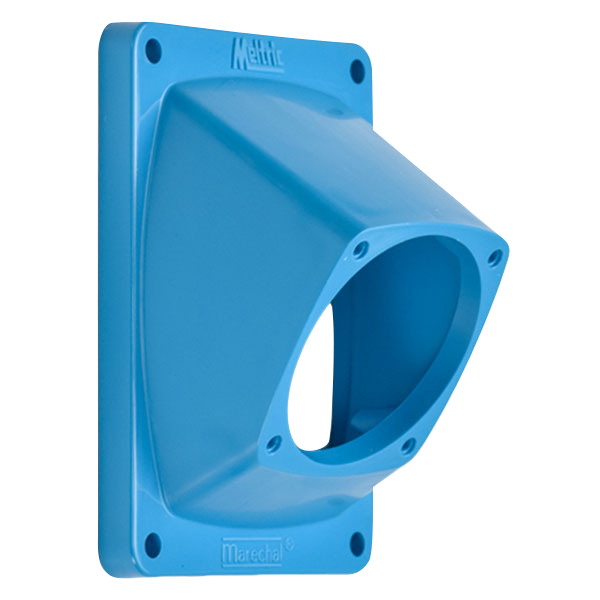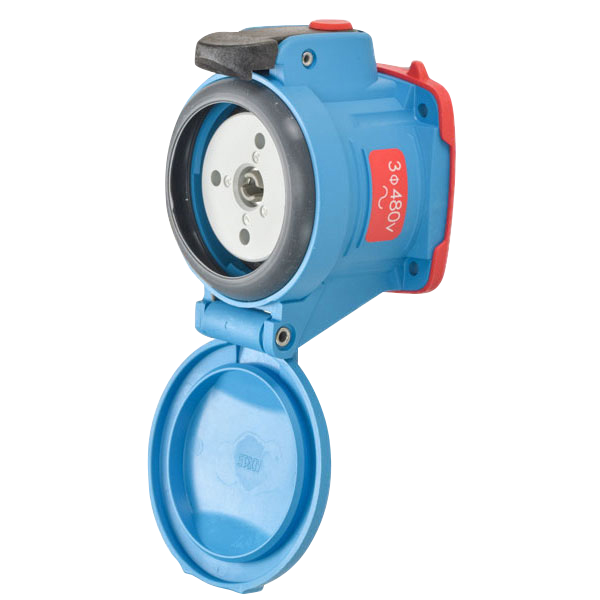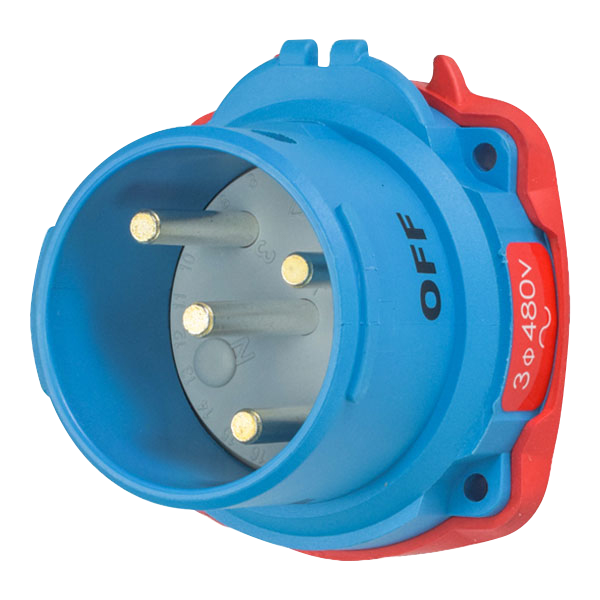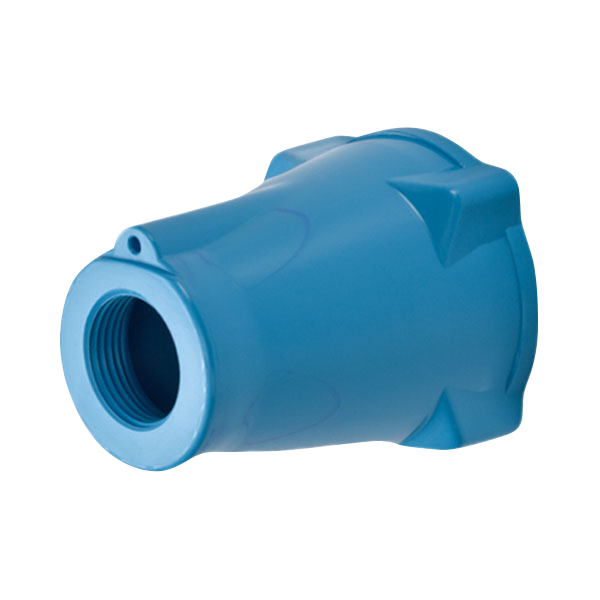# Part Number Guide

MELTRIC products are ordered and assembled in a modular fashion. Customers should select the desired male inlet and female receptacle part numbers. Then matching accessories such as handles, angles and junction boxes should be identified and added to the order to create plugs, connectors or other configurations. This modular system allows MELTRIC to build and ship product to customer specifications in a very short time - 90% of orders ship by the next business day.

## How to Order

### Accessory (Angle)### Receptacle### Inlet### Accessory (Handle)# Inlet/Receptacle Part Number Breakdown

## Example: Receptacle

63 6 4 04 3
MELTRIC Product Line Type and Casing Material AMP (A) Rating Form and Mounting Voltage Polarization Phasing
Position Voltage Hz
PN/PN7c DSN DXN Female AC
01 = Blue Poly 1 = 20 1 = 20 4 = Receptacle 01 = 220/250 50 2 = 2P+G AC
380/440
09 = Blue Metal 3 = 30 3 = 30   02 = 20/24 60 3 = 3P+G AC
PN12c 6 = 60 6 = 60 Male 03 = 110/130 50 5 = 1P+N+G AC
190/230
DN     8 = Inlet 04 = 255/277 60 6 = 2P+N+G AC
440/480
19 = Blue Metal DS DX   06 = 25/28 50 7 = 3P+N+G AC
39 = Blue Metal 1 = 20 2 = 20*   07 = 110/125 60 A* = 2P AC
220/250
3 = 30 3 = 30*   08 = 20/24 50 B* = 3P AC
DXN 6 = 60/100 6 = 60* DX, DXN37c, PXN12c, SPeX Only 09 = 480/500 50 C* = 3P+N AC
22 = Black Poly 9 = 100 9 = 100*   10 = 110/130 DC D* = 1P+N AC
2 = 200*   Female 11 = 115/127 400 G* = 2P+N DC
200/220
DX     0 = Receptacle on box 12 = 115/127 200
*
For 50V or less only
200/220
26 = Gray Metal DB PF 3 = Connector 13 = 40/48 50
3 = 30* 3 = 300* 4 = Receptacle 14 = 347/600 60
DS 6 = 60* 4 = 400*   16 = 120/127 60
208/220
33 = Blue Poly 9 = 100* 6 = 600* Male 17* = 110/125 60
220/250
35 = Black Poly     1 = Plug 18* = 347/600 60
37 = Blue Metal DR PFQ 6 = Inlet on Box 19 = 380/400 50
660/690
1 = 30 3 = 300*   20 = 220/250 DC
DR 3 = 50     22 = 577/1000 50
31 = Blue Poly 6 = 100 DN   23* = 120/127 60
39 = Blue Metal 208/220
9 = 150 1 = 20* (DN9)   24* = 255/277 60
440/480
2 = 250* 6 = 20* (DN20)   * For DS100C and DR devices
PF
49 = Gray Metal PN
N = 20 (IP66+IP67)
PFQ S = 20 (IP54/IP55)
47 = Gray Metal 2 = 20
3 = 30
DSN
63 = Blue Poly *Available in metal only
65 = Black Poly
Note: This is the Keying Standard for
MELTRIC products. Suffixes are available to accommodate non-interchangeable devices
of the same size at the same voltage. Please ask for more details.
DB
89 = Blue Metal
Note: On metal devices, plugs are not painted, receptacles are painted.